# MCQs on Control Systems

##### Page 15 of 23. Go to page 1 2 3 4 5 6 7 8 9 10 11 12 13 14 15 16 17 18 19 20 21 22 23
01․ A first order control system is initially at 0 V and subjected to a sudden input at t=0. Its output reaches 1.1 V in 4 sec and eventually reaches steady state of 2 V. Find the time constant?
4 sec
5 sec
2 sec
6 sec

For first order system, output c(t) = k (1-e-(t/τ)) At t = 4 sec, 1.1 = 2 (1-e-(4/τ)) 2*e-(4/τ) = 0.9 e-(4/τ) = 0.45 ln(e-(4/τ)) = ln(0.45) -4/τ = -0.8 Time constant τ = 5 sec

02․ A second order control system has a transfer function 16/(s² + 4s + 16). What is the time for the first overshoot?
2π/√3 sec
π/√3 sec
π/2√3 sec
π/4√3 sec

Given transfer function = 16/(s² + 4s + 16) By comparing standard second order equation, natural frequency ωn = 4 rad/s damping ratio ξ = 0.5 Peak time = nπ/ωd Where, n = overshoot number ωd = damped frequency = ωn*√(1-ξ²) Peak time = π/(4*√(1-0.5²) = π/2√3 sec

03․ Two identical first order systems have been cascaded non interactively. The unit step response of the systems will be
Over damped
under damped
critical damped
un damped

First order system transfer function = R/(RCS+1) When two identical first order systems have been cascaded non interactively, the transfer function = R/(RCS+1)² For the given transfer function, the roots are repeated. Therefore, response of the system is critical damped.

04․ A second order control system has a transfer function 16/(s² + 4s + 16). Find the settling time for 2% tolerance?
10 sec
5 sec
4 sec
2 sec

Given transfer function = 16/(s² + 4s + 16) The standard second order expression in terms of damping ratio (ξ) and natural frequency (ωn) is as follows. C(s)/R(s) = (ω²n)/(s² + 2ζ(ωn)s + ω²n) Therefore, natural frequency ωn = 4 rad/s damping ratio ξ = 0.5 Settling time for 2% tolerance = 4/(ξωn) = 4/(4*0.5) = 2 sec

05․ Settling time for 5% tolerance band is -------------
3T.
4T.
5T.
2T.

Settling time is the time taken by the response of the system to reach and stay within the limits of the tolerance band. For 2% tolerance band, settling time = 4/(ξ*ωn) = 4T. For 5% tolerance band, settling time = 3/(ξ*ωn) = 3T.

06․ The optimum values of damping ratio is --------------
0.9 to 1.1
0.3 to 5
0.3 to 0.7
0 to 2

Damping ratio ξ = Actual damping/ Critical damping. Most of the control systems are designed for damping ratio 'ξ' value less than 1. Because the response can be analysed using more number of performance specifications. The optimum value of damping ratio is 0.3 to 0.7.

07․ For the polynomial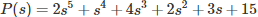, number of roots which lie in the right half of s-plane is
4
2
3
1

Applying Routh-Hurwitz criterion as shown below,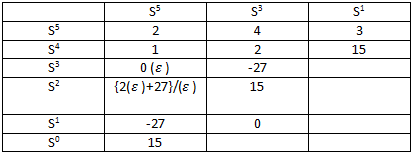We observe that the sign of the first column changes twice, so the number of roots on the right hand side of S plane = 2.

08․ For the polynomial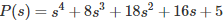, find the number of roots which lie on the right half of s-plane?
1
2
4
0

Applying Routh-Hurwitz criterion as shown below,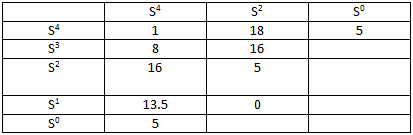We observe that the sign of the first column does not change, so the number of roots on the right hand side of S plane = 0.

09․ The system having characteristic equation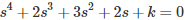is used to be an oscillator. What is the value of k?
1
2
3
4

Applying Routh-Hurwitz criterion as shown below,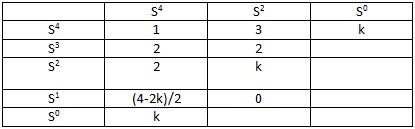For stability of the system, 1. (4-2k)/2 > 0 and 2. k > 0 k < 2 Therefore, 0 < k < 2 Marginal value of k = 2.

10․ The unit step response of the system is 1 - e-t(1+t). Which is the system?
unstable
stable
oscillatory
critically stable

Step response = 1 - e-t(1+t) d/dt(step response) = Impulse response L( Impulse response) = Transfer function Transfer function = 1/(s+1)² The stability of the LTI system may be defined as "when the system is subjected to bounded input the output should be bounded". Therefore, given system is absolutely stable.

<<<1314151617>>>